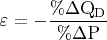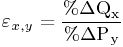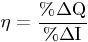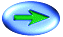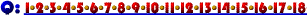Elasticity Multiple Choice Practice Questions
None of the elasticity questions require that you use the more complex arc elasticity formula; though if your prof expects you to know it, you should practice it. The questions are meant to be answered using the simple elasticity formulas.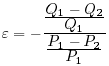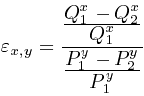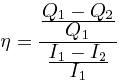On several of the questions you will find that you can work with the general formulas of the type: